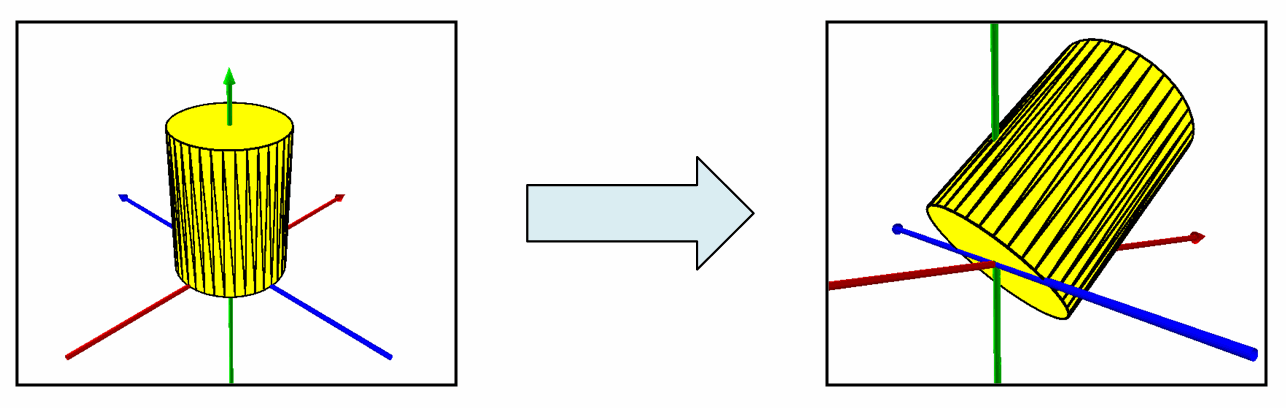ArcObjects Library Reference (Geometry)

# ITransform3D.RotateVector3D Method

Rotates the object about axis defined by the specified vector through an angle measured in radians.

```[Visual Basic .NET]
Public Sub RotateVector3D ( _
ByVal axis As IVector3D, _
ByVal rotationAngle As Double _
)```
```[C#]
public void RotateVector3D (
IVector3D axis,
double rotationAngle
);```
```[C++]
HRESULT RotateVector3D(
IVector3D* axis,
double rotationAngle
);```
```[C++]
Parameters
axis
axis is a parameter of type IVector3D
rotationAngle
rotationAngle is a parameter of type double
```

#### Product Availability

Available with ArcGIS Engine, ArcGIS Desktop, and ArcGIS Server.

#### Remarks

The angle of rotation must be in radians. To convert to radians from decimal degrees, multiply by PI/180.

[C#]public static void TransformMultiPatchGeometry()

{

const double DegreesOfRotation = 45;

//Transform3D: Cylinder Rotated Around An Axis Via RotateVector3D()

//Construct A Vector3D Corresponding To The Desired Axis Of Rotation

IVector3D axisOfRotationVector3D = ConstructVector3D(0, 10, 0);

//Obtain Angle Of Rotation In Radians

IGeometry geometry = GetMultiPatchGeometry();

ITransform3D transform3D = geometry as ITransform3D;

}

private static IVector3D ConstructVector3D(double xComponent, double yComponent, double zComponent)

{

IVector3D vector3D = new Vector3DClass();

vector3D.SetComponents(xComponent, yComponent, zComponent);

return vector3D;

}

{

return decimalDegrees * (Math.PI / 180);

}